Students can download 4th Maths Term 1 Chapter 1 Geometry Ex 1.5 Questions and Answers, Notes, Samacheer Kalvi 4th Maths Guide Pdf helps you to revise the complete Tamilnadu State Board New Syllabus, helps students complete homework assignments and to score high marks in board exams.

## Tamilnadu Samacheer Kalvi 4th Maths Solutions Term 1 Chapter 1 Geometry Ex 1.5

A. Find the perimeter of the following figures

i.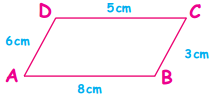Perimeter = 8 + 3 + 5 + 6
= 22 cmii.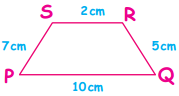Perimeter = 10 + 5 + 2 + 7
= 24 cm

iii.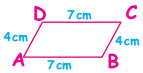Perimeter = 7 + 4 + 7 + 4
= 22 cmiv.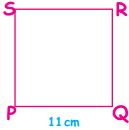Perimeter = 11 + 11 + 11 + 11
= 44 cm

v.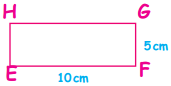Perimeter = 10 + 5 + 10 + 5
= 30 cmB. Solve the following

Question 1.
A side of a square-shaped sandbox in Gandhi Park measures 30 cm. Determine the perimeter of the sand box.
Side of a sand box = 30cm
Perimeter = 30 + 30 + 30 + 30
= 120 cm

Question 2.
Find the perimeter of a rectangle, whose sides are 12 cm and 8 cm.
Side of a rectangle are 12 cm and 8 cm.
Perimeter = 12 + 8 + 12 + 8
= 40cmQuestion 3.
Find the perimeter of the triangle, whose sides are 13 cm, 5 cm and 14 cm.
Length of sides are 13cm, 5cm and 14cm
Perimeter = 13 + 5 + 14
= 32cm

Question 4.
The adjacent sides of a parallelogram are 6 cm and 7 cm. What is the perimeter of the parallelogram?